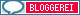Mar 03

## exercise – search-mechanism II

What is the output of the following code?

Pattern p = Pattern.compile(“\\d*” );
Matcher m = p.matcher(“Test4u21″);
while(m.find()) {
System.out.println(m.start() + ” ” +m.group());

Tagged with:
Mar 02

## exercise – search-mechanism

What is the output of the following code?

Pattern p = Pattern.compile(“\\s”);

Matcher m = p.matcher(“This is an exercise”);

while (m.find())

System.out.println(m.start());

Tagged with:
Mar 01

## search-mechanism – searching by changeable variables

surely you can also search with changeable variables:

String a = “\\d”;
String b = “c2ckcckcx9ckckx7c”;
Pattern p = Pattern.compile(a);
Matcher m = p.matcher(b);
boolean c = false;
while (c=m.find())

System.out.println(m.start() +” “+m.group());

So all decimal values are searched in String b. Therefore the output is:

1 2
9 9
15 7

Tagged with:
Feb 28

## search-mechanism – (non) greedy operators – + – one or unlimited

Today the last example for (non) greedy operators:

Pattern p = Pattern.compile(“ckc.+”);
Matcher m = p.matcher(“c2ckcckcx9ckckx7c”);
boolean b = false;
while (b=m.find())

System.out.println(m.start() +” “+m.group());

‘.’ is still the replacement for one character, with ‘+’ we are searching for one or unlimited Strings.

Therefore the Output is:

2 ckcckcx9ckckx7c

So first of all no difference to ‘*?’.  Let’s have a look on the change to non-greedy:

Pattern p = Pattern.compile(“ckc.+?“);

Here the Output:

2 ckcc
10 ckck

For comparison, while using ‘*’ (zero, one or unlimited appearance) the non-greedy output would be:

2 ckc
5 ckc
10 ckc

Tagged with:
Feb 27

## search-mechanism – (non) greedy operators – ? – zero or one

After focusing yesterday on ‘*?’ I would like to explain ‘??’ and ‘+?’.

Let’s start today with ‘??’:

Pattern p = Pattern.compile(“ckc.?”);
Matcher m = p.matcher(“c2ckcckcx9ckckx7c”);
boolean b = false;
while (b=m.find())

System.out.println(m.start() +” “+m.group());

‘.’ is again the replacement for any other character, ‘?’ means that this String should be there zero or one times.

Therefore the Output is:

2 ckcc
10 ckck

After change to non-greedy:

Pattern p = Pattern.compile(“ckc.??“);

the output is:

2 ckc
5 ckc
10 ckc

Tagged with:
Feb 26

## search-mechanism – greedy vs non greedy

Today I would like to explain what are (non) greedy operators and explain how to distinguish. As usual, let’s start with an example:

Pattern p = Pattern.compile(“ckc.*”);
Matcher m = p.matcher(“c2ckcckcx9ckckx7c”);
boolean b = false;
while (b=m.find())

System.out.println(m.start() +” “+m.group());

As already explained is ‘.’ the replacement for any other character, with ‘*’ we are searching for zero, one or unlimited of the String ‘ckc’. In combination we are searching for the String ‘ckc’ + further characters due to ‘.’

Therefore the Output is:

2 ckcckcx9ckckx7c

So everything is given out, the operator is greedy. More or less the order was to give out the longest possible String. In order to change this and having a non-greedy operator, a ‘?’ is added:

Pattern p = Pattern.compile(“ckc.*?“);

Now the Output is:

2 ckc
5 ckc
10 ckc

Tagged with:
Feb 25

## search-mechanism – replacement for any possible character

‘.’ is the replacement for any other possible character.

Pattern p = Pattern.compile(“1.3″);
Matcher m = p.matcher(“123 13 12 1c3″);
boolean b = false;
while (b=m.find())

System.out.println(m.start() +” “+m.group());

Therefore the output is:

0 123
10 1c3

Tagged with:
Feb 24

## search-mechanism – different statements added together

As you might already know you can also put together the different statements, eg

Pattern p = Pattern.compile(“\\w\\w\\w([-\\s])\\w\\w”);
Matcher m = p.matcher(“ccc-ccccc”);
boolean b = false;
while (b=m.find())

System.out.println(m.start() +” “+m.group());

A substring will be searched which contains 3 chars and than exactly one whitespace or a ‘-’ and than again 2 chars.

Therefore the output is: 0 ccc-cc

Should be more than one whitespace or ‘-’ be allowed we need to adjust the statement:

Pattern p = Pattern.compile(“\\w\\w\\w([-\\s])*\\w\\w”);

Tagged with:
Feb 23

## search-mechanism – all signs after a special sign

In case you need to give out all signs after a given sign (in our case still ‘c’) there is also a solution for that:

Pattern p = Pattern.compile(“c([^,])*”);
Matcher m = p.matcher(“cccxcc”);
boolean b = false;

while (b=m.find())

System.out.println(m.start() +” “+m.group());

Therefore the output is:

0 cccxcc

Tagged with:
Feb 22

## search-mechanism – quantifier: one or unlimited

With ‘+’ we are searching for one or unlimited ‘c’:

Pattern p = Pattern.compile(“c+”);
Matcher m = p.matcher(“cccxcc”);
boolean b = false;
while (b=m.find())

System.out.println(m.start() +” “+m.group());

In contradiction to previous examples, if ‘c’ does not occur, nothing will be found. Therefore the Output is:

0 ccc
4 cc

Tagged with:Webbhotell